##Cyclic Quadrilateral property

Consider a cyclic quadrilateral q = DEFG inscribed in the circle c(O,r). The intersection points K, J of opposite sides of (q) define the polar line (a) of the point A, A being the intersection point of the diagonals DF, EG of q (see the file Polar2.html ). The circle (d) with diameter JK is orthogonal to the circumcircle c of q. Besides the middles X, Y of the diagonals of q and the center N of (d) are collinear.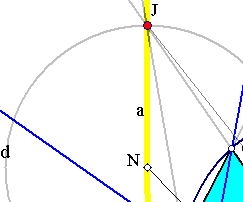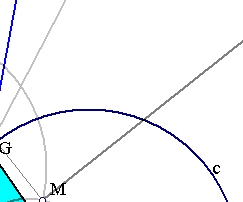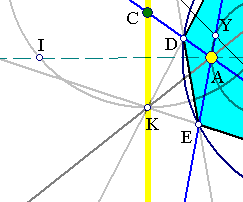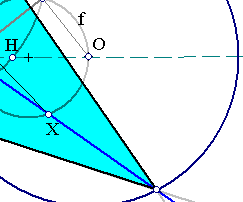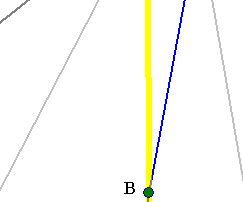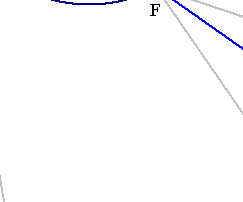In fact, by the properties discussed in Polar.html follows that the polar of point J is line [KA]. This line being orthogonal to OJ implies that its intersection point M with OJ is the inverse of J with respect to the inversion defined by the circle c. Thus circle (d) passes through the two inverse points J and M, hence is orthogonal to (c).
The figure has also another aspect. One can start with two points B, C on the polar line JK of point A. Then draw the secant lines [BEAG] and [CDAF] and build the quadrilateral q = DEFG etc. Then the circle (d) depends on the location of B, C on the polar of A, but it is always orthogonal to (c) as well as the circle (f), which is the inverse of the polar of A. Thus (d) is a member of the orthogonal bundle to the circles (c) and (f). In particular it passes through two fixed points H and I, which are the limit points of the bundle of circles generated by (c) and (f). Notice that the orthogonality of circles (c) and (d) implies that the polar of the center N of (d) is the radical axis of circles (c) and (d) and this line passes through A. Last assertion is a consequence of Newton's theorem. Notice that circle (f) is the locus of middles of all chords of (c) passing through A.
Document Cyclic.html discusses some further properties of the cyclic quadrilateral connected with circle (d).
Document Polar4.html discusses the same subject under a slightly different point of view.
Document Butterfly.html contains an application of these facts to a figure composed by chords of a circle.
For Newton's theorem on the middles of the diagonals of a complete quadrilateral see the file Newton.html .
See also the file OrthodiagonalFromCyclic.html discussing the derivation of an orthodiagonal quadrangle from a cyclic one, containing also a use of point H.
Related to this is the more general figure of a quadrangle inscribed in a conic. This is discussed in PascalOnQuadrangles.html .

Butterfly.html
CrossRatio0.html
Cyclic.html
Newton.html
OrthodiagonalFromCyclic.html S